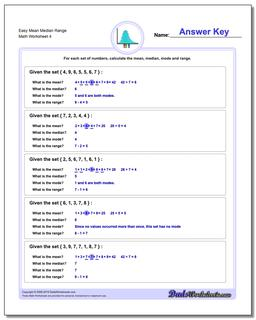# Math Worksheets: Mean, Median, Range: Mean, Median, Range: Easy Mean Median Range (Fourth Worksheet)## Easy Mean Median Range (Fourth Worksheet)

PropertyValue
DescriptionEasy Mean Median Range: Problem worksheets for finding the mean, median, mode and range given a set of numbers. These are basic problems suitable for "by-hand" calculations. The data in earlier problems are pre-sorted. (Fourth Worksheet)
Resource TypeWorksheet